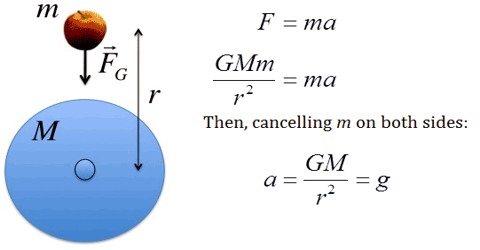# Magnitude of Acceleration of Gravity (g)

Magnitude of Acceleration of Gravity (g)

Acceleration of Gravity (g), its value is 9.8 m/s2 on Earth. That is to say, the acceleration of gravity on the surface of the earth at sea level is 9.8 m/s2. Near the Earth’s surface, the acceleration due to gravity is approximately constant. However, at large distances from the Earth, or around other planets or moons, the acceleration is different.

• Above the surface of the earth value of g decreases
• Inside the earth the value of g decreases
• Value of g decreases as one move from equator to the poles,
• Due to rotation of the earth, value of g decreases more in the equator regions compared to that in the poles.
• At the poles g = 9.832 ms-2, whereas at the equator, g = 9.790 ms-2. Value of g at Dhaka- 9.7835 ms-2: at Calcutta- 9.790 ms-2.
• The value of g above the sea-level and 450 latitude is taken as the standard which is 9.81 ms-2.
• Knowing the value of g, we can find the average density of the earth,